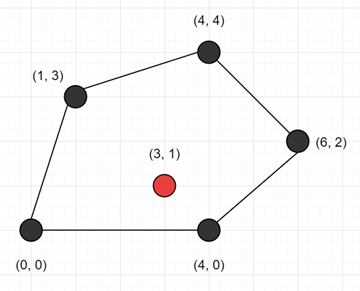# Program to check given point in inside or boundary of given polygon or not in python

Suppose we have a list of cartesian points [(x1, y1), (x2, y2), ..., (xn, yn)], that is representing a polygon, and also have two values x and y, we have to check whether (x, y) lies inside this polygon or on the boundary.

So, if the input is like points = [(0, 0), (1, 3), (4, 4), (6, 2), (4, 0)] pt = (3, 1)then the output will be True

To solve this, we will follow these steps −

• ans := False
• for i in range 0 to size of polygon - 1, do
• (x0, y0) := polygon[i]
• (x1, y1) := polygon[(i + 1) mod size of polygon]
• if pt is not in range minimum of y0, y1 and maximum of y0, y1, then
• go for next iteration
• if pt < minimum of x0 and x1, then
• go for next iteration
• cur_x := x0 if x0 is same as x1 otherwise x0 + (pt - y0) *(x1 - x0) /(y1 - y0)
• ans := ans XOR (1 when pt > cur_x is true, otherwise 0)
• return ans

Let us see the following implementation to get better understanding −

## Example

Live Demo

class Solution:
def solve(self, polygon, pt):
ans = False
for i in range(len(polygon)):
x0, y0 = polygon[i]
x1, y1 = polygon[(i + 1) % len(polygon)]
if not min(y0, y1) < pt <= max(y0, y1):
continue
if pt < min(x0, x1):
continue
cur_x = x0 if x0 == x1 else x0 + (pt - y0) * (x1 - x0) / (y1 - y0)
ans ^= pt > cur_x
return ans

ob = Solution()
points = [(0, 0), (1, 3), (4, 4), (6, 2), (4, 0)]
pt = (3, 1)
print(ob.solve(points, pt))

## Input

[(0, 0), (1, 3), (4, 4), (6, 2), (4, 0)], (3, 1)

## Output

True